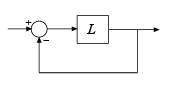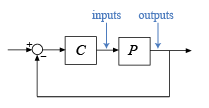# allmargin

Gain margin, phase margin, delay margin, and crossover frequencies

## Syntax

``S = allmargin(L)``
``S = allmargin(mag,phase,w,ts)``

## Description

example

````S = allmargin(L)` computes the gain margin, phase margin, delay margin, and the corresponding crossover frequencies for the SISO or MIMO negative feedback loop with open-loop response `L`. The negative feedback loop is computed as `feedback(L,eye(M))`, where `M` is the number of inputs and outputs in `L`.For a MIMO system, `allmargin` returns loop-at-a-time stability margins for the negative-feedback closed loop system. Use `allmargin` to find classical margins of any SISO or MIMO model, including models with delays.```

example

````S = allmargin(mag,phase,w,ts)` computes the stability margins from the frequency response data `mag`, `phase`, `w`, and the sample time, `ts`.```

## Examples

collapse all

For this example, consider a SISO open-loop transfer function `L` given by,

`$L\phantom{\rule{0.2777777777777778em}{0ex}}=\phantom{\rule{0.2222222222222222em}{0ex}}\frac{25}{{s}^{3}+10{s}^{2}+10s+10}$`

`L = tf(25,[1 10 10 10]);`

Find the stability margins of `L`.

`S = allmargin(L)`
```S = struct with fields: GainMargin: 3.6000 GMFrequency: 3.1623 PhaseMargin: 29.1104 PMFrequency: 1.7844 DelayMargin: 0.2847 DMFrequency: 1.7844 Stable: 1 ```

The output `S` is a structure with the classical margins and their respective crossover frequencies of the negative feedback loop of `L`.

For this example, consider a MIMO state-space model `L` with 2 inputs and 2 outputs.

`load('mimoStateSpaceModel.mat','L')`

Find the classical margins for the MIMO system.

`S = allmargin(L)`
```S=2×1 struct array with fields: GainMargin GMFrequency PhaseMargin PMFrequency DelayMargin DMFrequency Stable ```

The output `S` is a 2-by-1 structure array of classical margins and their respective crossover frequencies. For instance, `S(1)` refers to the stability margins of the first I/O feedback channel with all other loops closed.

For this example, load `invertedPendulumArray.mat`, which contains a 3-by-3 array of inverted pendulum SISO models. The mass of the pendulum varies as you move from model to model along a single column of `sys`, and the length of the pendulum varies as you move along a single row. The mass values used are 100g, 200g and 300g, and the pendulum lengths used are 3m, 2m and 1m respectively.

`$\begin{array}{cccc}& Column\phantom{\rule{0.5em}{0ex}}1& Column\phantom{\rule{0.5em}{0ex}}2& Column\phantom{\rule{0.5em}{0ex}}3\\ Row\phantom{\rule{0.5em}{0ex}}1& 100g,3m& 100g,2m& 100g,1m\\ Row\phantom{\rule{0.5em}{0ex}}2& 200g,3m& 200g,2m& 200g,1m\\ Row\phantom{\rule{0.5em}{0ex}}3& 300g,3m& 300g,2m& 300g,1m\end{array}$`

```load('invertedPendulumArray.mat','sys'); size(sys)```
```3x3 array of transfer functions. Each model has 1 outputs and 1 inputs. ```

Find stability margins for all models in the array.

`S = allmargin(sys)`
```S=3×3 struct array with fields: GainMargin GMFrequency PhaseMargin PMFrequency DelayMargin DMFrequency Stable ```

`allmargin` returns a 3-by-3 structure array `S`, in which each entry is a structure containing the stability margins of the corresponding entry in `sys`. For instance, the stability margins of the model with 100g pendulum weight and 2m length is contained in `S(1,2)`.

For this example, load the frequency response data of an open loop system, consisting of magnitudes `m` and phase values `p` measured at the frequencies in `w`.

`load('openLoopFRData.mat','m','p','w','ts');`

Compute stability margins using the frequency response data.

`S = allmargin(m,p,w,ts)`
```S = struct with fields: GainMargin: 0.6249 GMFrequency: 1.2732 PhaseMargin: [-90.0000 48.9853] PMFrequency: [1.0000 1.5197] DelayMargin: [4.7124 0.5626] DMFrequency: [1.0000 1.5197] Stable: NaN ```

The output `S` is a structure with the classical margins and their respective crossover frequencies. Since `allmargin` cannot assess the stability for frequency response data models, `S.Stable = NaN`.

## Input Arguments

collapse all

Open-loop response, specified as a dynamic system model. `L` can be SISO or MIMO, as long as it has the same number of inputs and outputs. `allmargin` computes the classical stability margins for the negative-feedback closed-loop system `feedback(L,eye(M))`:To compute the stability margins of the positive feedback system `feedback(L,eye(M),+1)`, use `allmargin(-L)`.

When you have a controller `P` and a plant `C`, you can compute the stability margins for gain and phase variations at the plant inputs or outputs. From the following diagram:• To compute margins at the plant outputs, set ```L = P*C```.

• To compute margins at the plant inputs, set ```L = C*P```.

`L` can be continuous time or discrete time. If `L` is a generalized state-space model (`genss` or `uss`), then `allmargin` uses the current or nominal value of all control design blocks in `L`.

If `L` is a frequency-response data model (such as `frd`), then `allmargin` computes the margins at each frequency represented in the model. The function returns the margins at the frequency with the smallest stability margin.

If `L` is a model array, then `allmargin` computes margins for each model in the array.

Magnitude of the system response in absolute units, specified as a 3-D array. `mag` is an M-by-M-by-N array, where M is the number of inputs or outputs, and N is the number of frequency points. For more information on obtaining `mag`, see Obtain Magnitude and Phase Data and Magnitude and Phase of MIMO System.

Phase of the system response in degrees, specified as a 3-D array. `phase` is an M-by-M-by-N array, where M is the number of inputs or outputs, and N is the number of frequency points. For more information on obtaining `phase`, see Obtain Magnitude and Phase Data and Magnitude and Phase of MIMO System.

Frequencies at which the magnitude and phase values of system response are obtained, specified as a column vector. You can provide the frequency vector `w` in any units; `allmargin` returns frequencies in the same units. `allmargin` interpolates between frequency points to approximate the true stability margins.

Sample time, specified as an integer. `allmargin` uses `ts` to find the stability margins from frequency response data.

• For continuous-time models, set `ts = 0`.

• For discrete-time models, `ts` is a positive integer representing the sampling period. To denote a discrete-time model with unspecified sample time, set `ts = -1`.

## Output Arguments

collapse all

Gain, phase, and delay margins, returned as a structure array.

The output `S` is a structure with the following fields:

• `GMFrequency`: All -180° (modulo 360°) crossover frequencies in `rad/TimeUnit`, where `TimeUnit` is the time units, specified in the `TimeUnit` property of `L`.

• `GainMargin`: Corresponding gain margins, defined as `1/G`, where `G` is the gain at the -180° crossover frequency. Gain margins are in absolute units.

• `PMFrequency`: All 0-dB crossover frequencies in `rad/TimeUnit`, where `TimeUnit` is the time units, specified in the `TimeUnit` property of `L`.

• `PhaseMargin`: Corresponding phase margins in degrees.

• `DMFrequency` and `DelayMargin`: `DelayMargin` is the maximum amount of delay that the system can tolerate before it loses stability. `DMFrequency` contains critical frequencies corresponding to the delay margins. Delay margins are specified in the time units of the system for continuous-time systems and multiples of the sample time for discrete-time systems.

• `Stable`: `1` if the nominal closed-loop system is stable, `0` if unstable, and `NaN` if stability cannot be assessed. In general, `allmargin` cannot assess the stability of an `frd` system.

When `L` is an M-by-M MIMO system, `S` is an M-by-1 structure array. For instance, `S(j)` gives the stability margins for the j-th feedback channel with all other loops closed (one-loop-at-a-time margins).

## Tips

• `allmargin` assumes that the system with open-loop response `L` is a negative-feedback system. To compute the classical stability margins of the positive feedback system `feedback(L,eye(M),+1)`, use `allmargin(-L)`.

• To compute classical margins for a system modeled in Simulink®, first linearize the model to obtain the open-loop response at a particular operating point. Then, use `allmargin` to compute classical stability margins for the linearized system. For more information, see Stability Margins of a Simulink Model (Robust Control Toolbox).